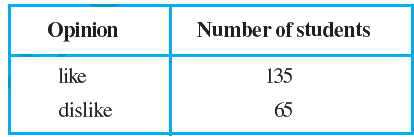# Ex.15.1 Q7 Probability Solution - NCERT Maths Class 9

Go back to  'Ex.15.1'

## Question

To know the opinion of the students about the subject statistics, a survey of $$200$$ students was conducted. The data is recorded in the following table.Find the probability that a student chosen at random

(i) likes statistics,

(ii) does not like it.

Video Solution
Probability
Ex exercise-15-1 | Question 7

## Text Solution

What is known?

Number of students who like statistics and who do not like statistics.

What is the unknown?

Probability of number of students who like statistics and who do not like statistics.

Reasoning:

The empirical probability $$P(E)$$ of an event E happening, is given by:

\begin{align}{P}({E})=\frac{ \begin{pmatrix} \text { Number of trials in which }\\ \text{the event happened } \end{pmatrix} }{ \text { The total number of trials } }\end{align}

Use probability to derive the solution where

Probability (students like/dislike statistics)

\begin{align}=\frac{ \begin{pmatrix} \text { Number of students who } \\ \text{like or dislike statistics } \end{pmatrix} }{\text { Total number of students }}\end{align}

Steps:

Total no of students $$= 200$$

(i) Likes statistics

Probability students like statistics

\begin{align}&\text{ = }\frac{\text{No of students like statistics }}{\text{ Total no of students}} \\ & \text{= }\frac{135}{200}\text{=}\frac{27}{40} \end{align}

(ii) Does not likes statistics

Probability students dislike statistics

\begin{align}&\text{ = }\frac{\text{No of students dislike statistics }}{\text{ Total no of students}} \\ & \text{= }\frac{65}{200}\text{=}\frac{13}{40} \end{align}

Learn from the best math teachers and top your exams

• Live one on one classroom and doubt clearing
• Practice worksheets in and after class for conceptual clarity
• Personalized curriculum to keep up with school